« Behaviour factor q Dynamic analysis και natural periods of structure » Design spectrum of horizontal seismic actions Design spectrum of horizontal seismic actions [EC8, §3.2.2.5 & §3.2.1(3)] For the horizontal components of the seismic action the design spectrum, Sd(T), is defined by the following expressions: 0 ≤ T < TB: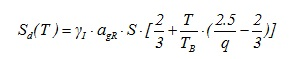TB ≤ T < TC: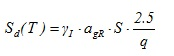TC ≤ T < TD: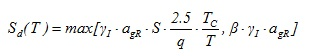TD ≤ T: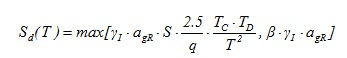The recommended value, provided by the EC8, for β is 0.20. Example : for importance class II → γI = 1.0, soil type B → S = 1.2, seismic zone withagR = 0.24 and q = 4.5, for values of Τ 0.1, 0.25, 1.0 and 3.0, the corresponding values of Sd are 0.171g, 0.16g, 0.08g and0.048g.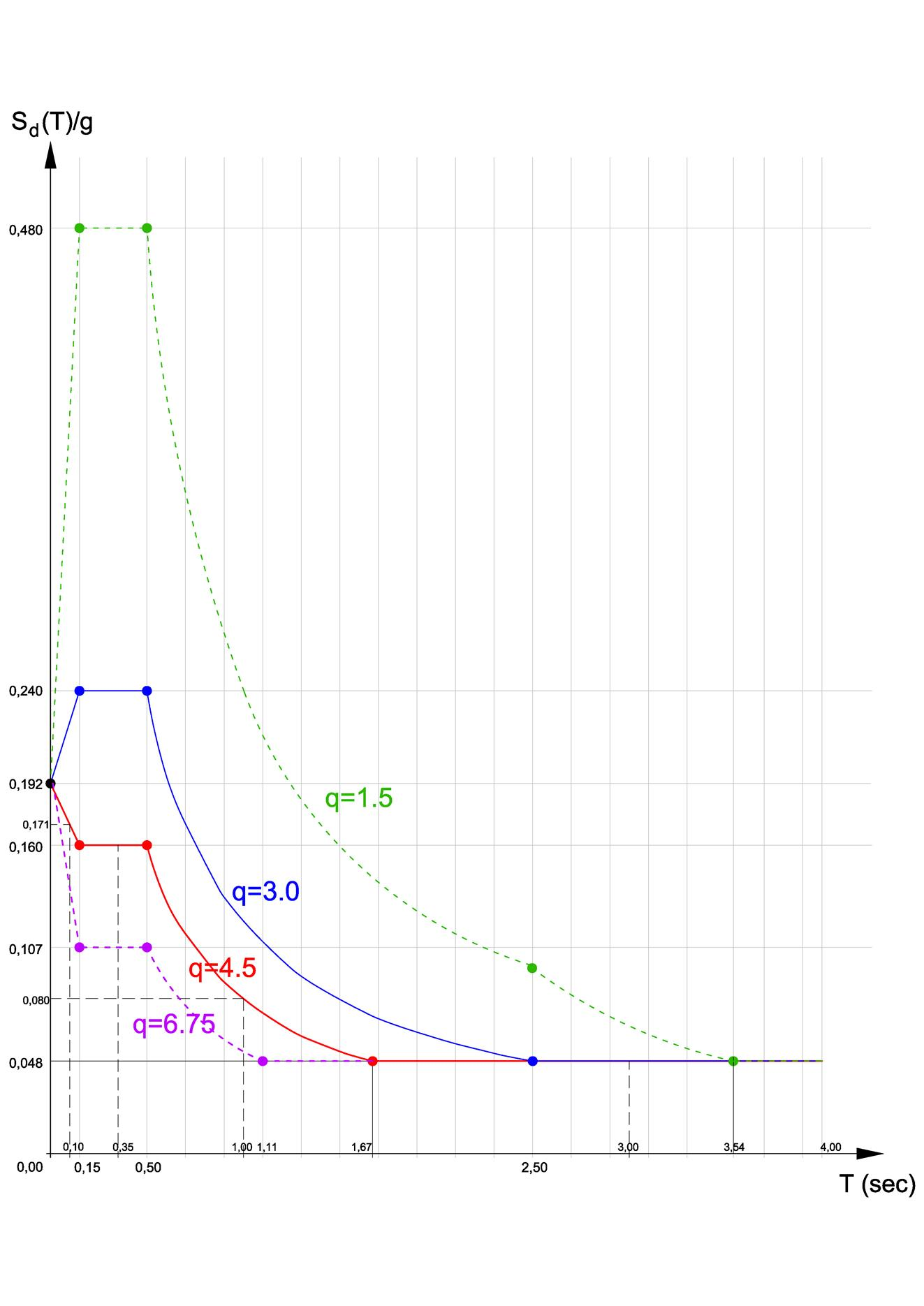Figure 6.1.6: Design spectrum of horizontal actions (a) for q = 4.5, (b) for q = 3.0 and (c) for q = 1.5.Seismic zone with agR = 0.24, TD = 2.5, soil type B, importance class ΙΙ Figure 6.1.6: Design spectrum of horizontal actions (a) for q = 4.5, (b) for q = 3.0 and (c) for q = 1.5.Seismic zone with agR = 0.24, TD = 2.5, soil type B, importance class ΙΙ Setting agR = 0.24, S = 1.2, γ I = 1.0 yields γ I · agR · S = 1.0 ·0.24·1,2 = 0.288. The expressions for four different values of q are the following: For q = 6.75 yields 2.5/q = 2.5/6.75 = 0.3703 0 ≤ T < 0.15: Sd (0) = 0.288·2/3 = 0.192, Sd(0.15) = 0.288·2.5/6.75 = 0.107 → straightline segment between points (0.0, 0.192), (0.15, 0.0107) Sd(T) = 0.288·[2/3+T/0.15· (2.5/6.75-2/3)] = 0.192-0.569T 0.15 ≤ T < 0.50: curve Sd(T) = 0.107 → straight line segment in the whole range 0.50 ≤ T < 2.50: curve Sd(T) = max ( γ I · agR · S · 2.5/q[TC/T], β · γ I · agR) = max(0.107 · 0.50/T, 0.048) curvature point at Τ = 1.11 → straight line segment after Τ = 1.11 2.50 ≤ T < 4.0: curve Sd(T) = max( max ( γ I · agR · S · 2.5/q[TCTD/T2], β · γ I · agR) = max(0.107 · 1.25/T2, 0.048) curvature point at Τ = 1.67 → straight line segment in the whole range For q = 4.5 yields 2.5/q = 2.5/4.5 = 25/45 = 0.555555556 0 ≤ T < 0.15: Sd (0) = 0.288 · 2/3 = 0.192, Sd(0.15) = 0.288 · 25/45 = 0.16 → straight line segment between points (0.0, 0.192), (0.15, 0.16) Sd(T) = 0.288· [2/3+T/0.15 · (2.5/4.5-2/3)] = 0.192-0.213T 0.15 ≤ T < 0.50: curve Sd(T) = 0.16 → straight line segment in the whole range at 0.16 0.50 ≤ T < 2.50: curve Sd(T) = max ( γ I · agR · S · 2.5/q[TC/T], β · γ I · agR) = max(0.16 · 0.50/T, 0.048) curvature point at Τ = 1.67 → straight line segment after Τ = 1.67 2.50 ≤ T < 4.0: curve Sd(T) = max( max ( γ I · agR · S · 2.5/q[TCTD/T2], β · γ I · agR) = max(0.16 · 1.25/T2, 0.048) curvature point at Τ = 2.04 → straight line segment in the whole range For q = 3.0 yields 2.5/q = 2.5/3.0 = 25/30 = 0.83333333333333 0 ≤ T < 0.15: Sd (0) = 0.288 · 2/3 = 0.192, Sd(0.15) = 0.288 · 0.83333333 = 0.24 → straight line segment between points (0.0, 0.192), (0.15, 0.24) Sd(T) = 0.288·[2/3+T/0.15 ·(2.5/3.0-2/3)] = 0.192+0.32T 0.15 ≤ T < 0.50: curve Sd(T) = 0.24 → straight line segment in the whole range 0.50 ≤ T < 2.50: curve Sd(T) = max ( γ I · agR · S · 2.5/q[TC/T], β · γ I · agR) = max(0.24 · 0.50/T, 0.048) curvature point at Τ = 2.5 → straight line segment does not exist 2.50 ≤ T < 4.0: curve Sd(T) = max( max ( γ I · agR · S · 2.5/q[TCTD/T2], β · γ I · agR) = max(0.24 · 1.25/T2, 0.048) curvature point at Τ = 2.5 → straight line segment in the whole range at 0.048 For q = 1.5 yields 2.5/q = 2.5/1.5 = 25/15 = 0.83333333333333 0 ≤ T < 0.15: Sd (0) = 0.288·2/3 = 0.192, Sd(0.15) = 0.288·1.66666666666667 = 0.48 → straightline segment between points (0.0, 0.192), (0.15, 0.48) Sd(T) = 0.288·[2/3+T/0.15· (2.5/1.5-2/3)] = 0.192+1.92T 0.15 ≤ T < 0.50: curve Sd(T) = 0.48 → straight line segment in the whole range 0.50 ≤ T < 2.50: curve Sd(T) = max ( γ I · agR · S · 2.5/q[TC/T], β · γ I · agR) = max(0.48 · 0.50/T, 0.048) curvature point at Τ = 5.0, → straight line segment does not exist 2.50 ≤ T < 4.0: curve Sd(T) = max( max ( γ I · agR · S · 2.5/q[TCTD/T2], β · γ I · agR) = max(0.48 · 1.25/T2, 0.048) curvature point at Τ = 3.54 → straight line segment between Τ = 3.54 and Τ = 4.0 at 0.048 Design spectrum of vertical seismic actions [EC8, §3.2.2.5(5)] The design of buildings usually does not account for the vertical component of the seismic action (see §6.6.2), but when it does it shall satisfy the following conditions: The design spectrum of §6.1.6 shall be adopted, replacing ag with avg = 0.9ag [EC8, §3.2.3, table 3.4] Soil factor S shall be taken equal to 1.0 Behaviour factor q shall be taken equal or less than 1.5 When demanding conditions occur, e.g. in multistorey planted columns, it is imposed for the vertical accelerations to be taken into account and maybe even a uniform value q = 1.5 should be considered for the whole structure, used for the calculation of the horizontal accelerations. In a serious case, it is useful to also consider the structure without the diaphragmatic behaviour of floors, at least at nodes where particularities exist, so that an exact value of the vertical seismic acceleration is obtained at the critical nodes. For practical and safety reasons, the vertical acceleration of critical nodes may be taken equal to the maximum vertical accelerationaV occurred at any node. This maximum vertical acceleration is equal to aV,max = 0.9·γI·agR·2.5/1.5 → aV,max = 1.5·γI ·agR. « Behaviour factor q Dynamic analysis και natural periods of structure »